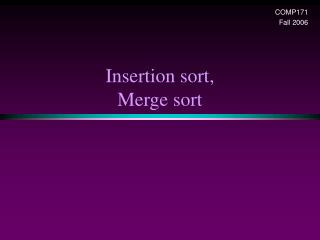DownloadDownload PresentationInsertion sort, Merge sort

# Insertion sort, Merge sort

Download Presentation## Insertion sort, Merge sort

- - - - - - - - - - - - - - - - - - - - - - - - - - - E N D - - - - - - - - - - - - - - - - - - - - - - - - - - -
##### Presentation Transcript

1. COMP171 Fall 2006 Insertion sort,Merge sort

2. Insertion sort 1) Initially p = 1 2) Let the first p elements be sorted. 3) Insert the (p+1)th element properly in the list so that now p+1 elements are sorted. 4) increment p and go to step (3)

3. Insertion Sort

4. Insertion Sort... see applet http://www.cis.upenn.edu/~matuszek/cse121-2003/Applets/Chap03/Insertion/InsertSort.html • Consists of N - 1 passes • For pass p = 1 through N - 1, ensures that the elements in positions 0 through p are in sorted order • elements in positions 0 through p - 1 are already sorted • move the element in position p left until its correct place is found among the first p + 1 elements

5. Extended Example To sort the following numbers in increasing order: 34 8 64 51 32 21 p = 1; tmp = 8; 34 > tmp, so second element a is set to 34: {8, 34}… We have reached the front of the list. Thus, 1st position a = tmp=8 After 1st pass: 8 34 64 51 32 21 (first 2 elements are sorted)

6. P = 2; tmp = 64; 34 < 64, so stop at 3rd position and set 3rd position = 64 After 2nd pass: 8 34 64 51 32 21 (first 3 elements are sorted) P = 3; tmp = 51; 51 < 64, so we have 8 34 64 64 32 21, 34 < 51, so stop at 2nd position, set 3rd position = tmp, After 3rd pass: 8 34 51 64 32 21 (first 4 elements are sorted) P = 4; tmp = 32, 32 < 64, so 8 34 51 64 64 21, 32 < 51, so 8 34 51 51 64 21, next 32 < 34, so 8 34 34, 51 64 21, next 32 > 8, so stop at 1st position and set 2nd position = 32, After 4th pass: 8 32 34 51 64 21 P = 5; tmp = 21, . . . After 5th pass: 8 21 32 34 51 64

7. Analysis: worst-case running time • Inner loop is executed p times, for each p=1..N-1  Overall: 1 + 2 + 3 + . . . + N-1 = …= O(N2) • Space requirement is O(?)

8. Analysis • The bound is tight (N2) • That is, there exists some input which actually uses (N2) time • Consider input as a reversed sorted list • When a[p] is inserted into the sorted a[0..p-1], we need to compare a[p] with all elements in a[0..p-1] and move each element one position to the right (i) steps • the total number of steps is (1N-1i) = (N(N-1)/2) = (N2)

9. Analysis: best case • The input is already sorted in increasing order • When inserting A[p] into the sorted A[0..p-1], only need to compare A[p] with A[p-1] and there is no data movement • For each iteration of the outer for-loop, the inner for-loop terminates after checking the loop condition once => O(N) time • If input is nearly sorted, insertion sort runs fast

10. Some Revision • How do we revise insertion sort, so that the insertion of a new element at the p-th index is done using binary search (since the sub-array A[0..p-1] is already sorted?) • Question: what is the worst case time complexity then? • Binary Search Code: (see next page) • Question: how to revise insertion sort to use this function?

11. Binary search code (from recursion lecture) // Searches an ordered array of integers using recursion int bsearchr(constint data[], // input: array int first, // input: lower bound int last, // input: upper bound int value // input: value to find )// output: index if found, otherwise return –1 { int middle = (first + last) / 2; if (data[middle] == value) return middle; else if (first >= last) return -1; else if (value < data[middle]) return bsearchr(data, first, middle-1, value); else return bsearchr(data, middle+1, last, value); }

12. New code for insertion sort: fill out the … part: { int tmp=a[p]; int index = bsearchr(…); for (j=p; … ; j--) …; a[j] = tmp; }